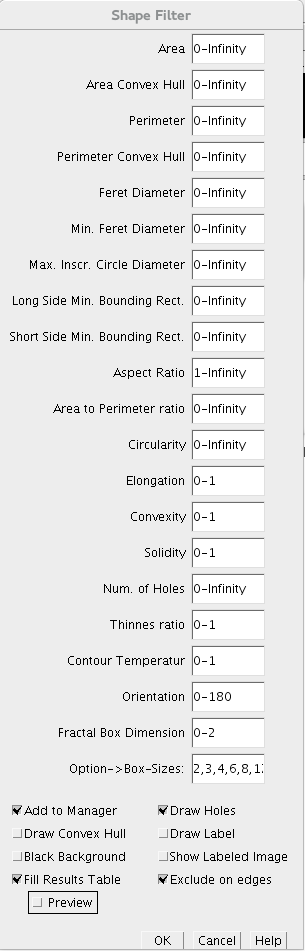# Shape FilterThe content of this page has not been vetted since shifting away from MediaWiki. If you’d like to help, check out the how to help guide!

# General Description

The ImageJ Shape Filter Plugin use the [ij-blob] library to characterize and filter objects in binary scenes by its shape. Therefore, several features are calculated as shown below.If you like to cite the Shape Filter plugin in a scientific publication, please cite:

doi:10.5334/jors.ae

## Shape Features

• Area ($$A$$): The area enclosed by the outer contour of an object.
• Area Convex Hull ($$C$$): The area enclosed by the convex hull of the outer contour of an object.
• Perimeter ($$P$$): The perimeter of the outer contour of an object.
• Perimeter Convex Hull ($$H$$): The perimeter of the convex hull of the particle.
• Feret Diameter: The maximum distance between the two parallel tangents touching the particle outline in all directions.
• Min. Feret Diameter: the minimum distance between the two parallel tangents touching the particle outline in all directions.
• Max. Inscr. Circle Diameter: The diameter of the maximum inscribed circle.
• Area eq. circle diameter: Equivalent circle diameter $$2\sqrt{\frac{A}{\pi}}$$
• Long Side Minimum Bounding Rectangle ($$L$$): The larger side of the minimum bounding rectangle.
• Short Side Minimum Bounding Rectangle ($$S$$): The smaller side of the minimum bounding rectangle.
• Aspect Ratio: Defined as $$L/S$$
• Area to Perimeter Ratio: Defined as $$A/P$$
• Circularity: Defined as $$P^{2}/A$$
• Elongation: Defined as $$1 - S/L$$
• Convexity: Defined as $$H/P$$
• Solidity: Defined as $$A/C$$
• Number of Holes: The number of holes inside an object.
• Thinnes Ratio: Inverse proportional to the circularity. Furthermore it normed. It is defined as $$4\pi A/P^{2}$$
• Contour Temperatur: It has a strong relationship to the fractal dimension, defined as $$\left(log_{2}\left(\frac{2P}{P-H}\right)\right)^{-1}$$
• Orientation: The orientation of the major axis from in grad (measured counter clockwise from the positive x axis).
• Fractal Box Dimension: Estimated fractal dimension by the box count algorithm. The default box-sizes are “2,3,4,6,8,12,16,32,64”.

# Installation

You could simply use our update site “Biomedgroup” to install the shape filter plugin.